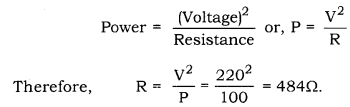# An electric lamp is marked 100W, 220V. It is used for 5 hours daily. Calculate. (i) its resistance while glowing

An electric lamp is marked 100W, 220V. It is used for 5 hours daily. Calculate.
(i) its resistance while glowing.
(ii) energy consumed in kwh per day.

(i) Resistance of a glowing lamp is related to its power and voltage as.Therefore, the resistance of the bulb when glowing is 484Q.

(ii) Power = 100W = 0.1 kw
Energy = Power × Time = 0.1 Kw × 5h = 0.5KWh
0.5 kwh is the amount of energy consumed by the bulb per day.